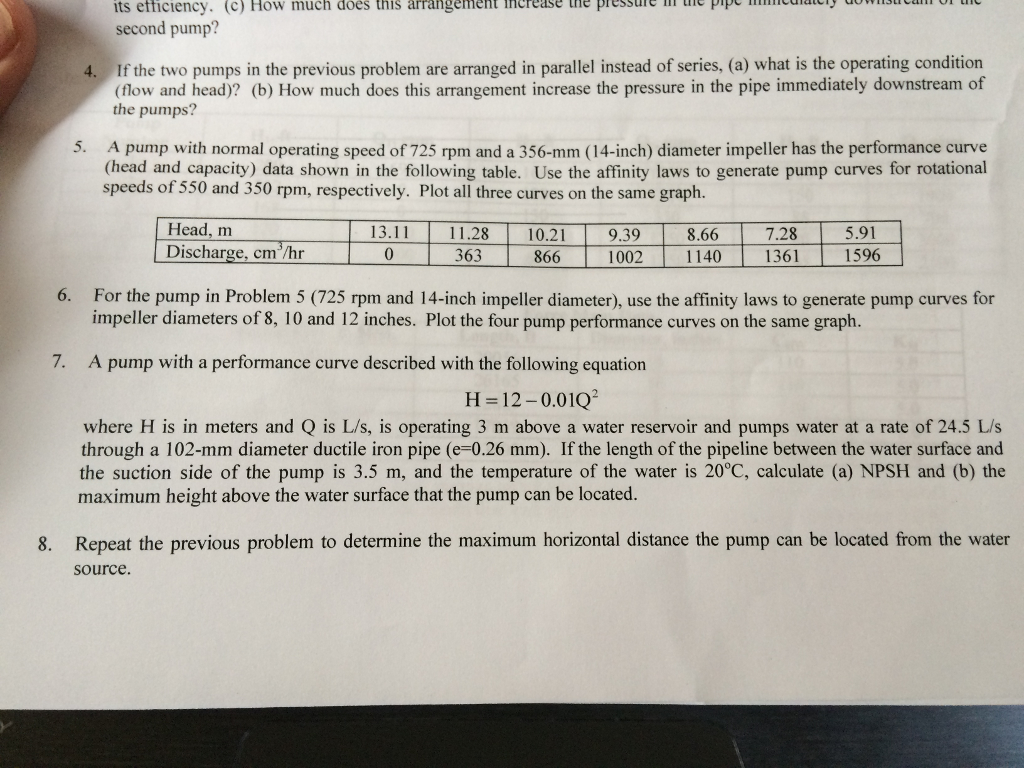# If the two pumps in the previous problem are arranged in parallel instead of series, (a) what is …I just need help with problems 7 and 8 thanks!

If the two pumps in the previous problem are arranged in parallel instead of series, (a) what is the operating condition (flow and head)? (b) How much does this arrangement increase the pressure in the pipe immediately downstream of the pumps? A pump with normal operating speed of 725 rpm and a 356-mm (14-inch) diameter impeller has the performance curve (head and capacity) data shown in the following table. Use the affinity laws to generate pump curves for rotational speeds of 550 and 350 rpm, respectively. Plot all three curves on the same graph. For the pump in Problem 5 (725 rpm and 14-inch impeller diameter), use the affinity laws to generate pump curves for impeller diameters of 8, 10 and 12 inches. Plot the four pump performance curves on the same graph. A pump with a performance curve described with the following equation H=12-0.01Q^2 where H is in meters and Q is L/s, is operating 3 m above a water reservoir and pumps water at a rate of 24.5 L/s through a 102-mm diameter ductile iron pipe (e=0.26 mm). If the length of the pipeline between the water surface and the suction side of the pump is 3.5 m, and the temperature of the water is 20 degree C, calculate (a) NPSH and (b) the maximum height above the water surface that the pump can be located. Repeat the previous problem to determine the maximum horizontal distance the pump can be located from the water Source.